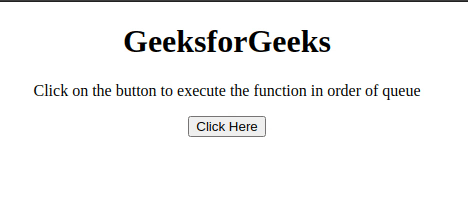# How to store JavaScript functions in a queue and execute in that order?

The task is to execute the functions in the order defined in the queue with the help of JavaScript. There are two approaches that are discussed below.

Approach 1: Declare the functions and use array push() method to push the functions in the array. Later traverse the array and execute the functions one by one.

• Example: This example implements the above approach.

 ` ` `<``html``> ` `    ``<``head``> ` `        ``<``title``> ` `            ``Store JavaScript functions in  ` `            ``a queue and execute in that order ` `        `` ` `    `` ` `    ``<``body` `style``=``"text-align: center;"``> ` `        ``<``h1``> ` `            ``GeeksforGeeks ` `        `` ` `        ``<``p` `id``=``"GFG_UP"``> ` `        ``<``button` `onclick``=``"myGFG()"``> ` `            ``Click Here ` `        `` ` `        ``<``p` `id``=``"GFG_DOWN"``> ` `        ``<``script``> ` `            ``var up = document.getElementById("GFG_UP"); ` `            ``up.innerHTML =  ` `              ``"Click on the button to execute "+ ` `              ``"the function in order of queue"; ` `            ``var down = document.getElementById("GFG_DOWN"); ` `            ``function myGFG() { ` `               `  `                ``// First function ` `                ``function function1() { ` `                    ``alert("First Function"); ` `                ``} ` `               `  `                ``// Second function ` `                ``function function2() { ` `                    ``alert("Second Function"); ` `                ``} ` `                ``var orderQueue = []; ` `               `  `                ``// Push them in queue ` `                ``orderQueue.push(function1); ` `                ``orderQueue.push(function2); ` `                ``while (orderQueue.length > 0) { ` `                    ``// Execute in order ` `                    ``orderQueue.shift()(); ` `                ``} ` `                ``down.innerHTML =  ` `                  ``"Functions executed in queue order"; ` `            ``} ` `        `` ` `    `` ` ` `

• Output:Approach 2:Declare the functions and use arrayindexing to assign the functions to index of the array in the order. Later traverse the array and execute the functions one by one.

• Example: This example implements the above approach.

 ` ` `<``html``> ` `    ``<``head``> ` `        ``<``title``> ` `            ``Store JavaScript functions in a  ` `            ``queue and execute in that order ` `        `` ` `    `` ` `    ``<``body` `style``=``"text-align: center;"``> ` `        ``<``h1``> ` `            ``GeeksforGeeks ` `        `` ` `        ``<``p` `id``=``"GFG_UP"``> ` `        ``<``button` `onclick``=``"myGFG()"``> ` `            ``Click Here ` `        `` ` `        ``<``p` `id``=``"GFG_DOWN"``> ` `        ``<``script``> ` `            ``var up = document.getElementById("GFG_UP"); ` `            ``up.innerHTML =  ` `              ``"Click on the button to execute "+ ` `              ``"the function in order of queue"; ` `            ``var down = document.getElementById("GFG_DOWN"); ` `            ``function myGFG() { ` `               `  `                ``// First function ` `                ``function function1() { ` `                    ``alert("First Function"); ` `                ``} ` `               `  `                ``// Second function ` `                ``function function2() { ` `                    ``alert("Second Function"); ` `                ``} ` `                ``var functions = new Array(); ` `                ``// Adding the functions in the order ` `                ``// in queue(array) ` `                ``functions = function1; ` `                ``functions = function2; ` `                ``for (var i = 0; i < ``functions.length``; i++) { ` `                   `  `                    ``// Executing them in order. ` `                    ``functions[i].call(); ` `                ``} ` `                ``down.innerHTML` `=  ` `                    ``"Functions executed in queue order"``; ` `            ``} ` `        `` ` `    `` ` ` `

• Output:My Personal Notes arrow_drop_upCheck out this Author's contributed articles.

If you like GeeksforGeeks and would like to contribute, you can also write an article using contribute.geeksforgeeks.org or mail your article to contribute@geeksforgeeks.org. See your article appearing on the GeeksforGeeks main page and help other Geeks.

Please Improve this article if you find anything incorrect by clicking on the "Improve Article" button below.